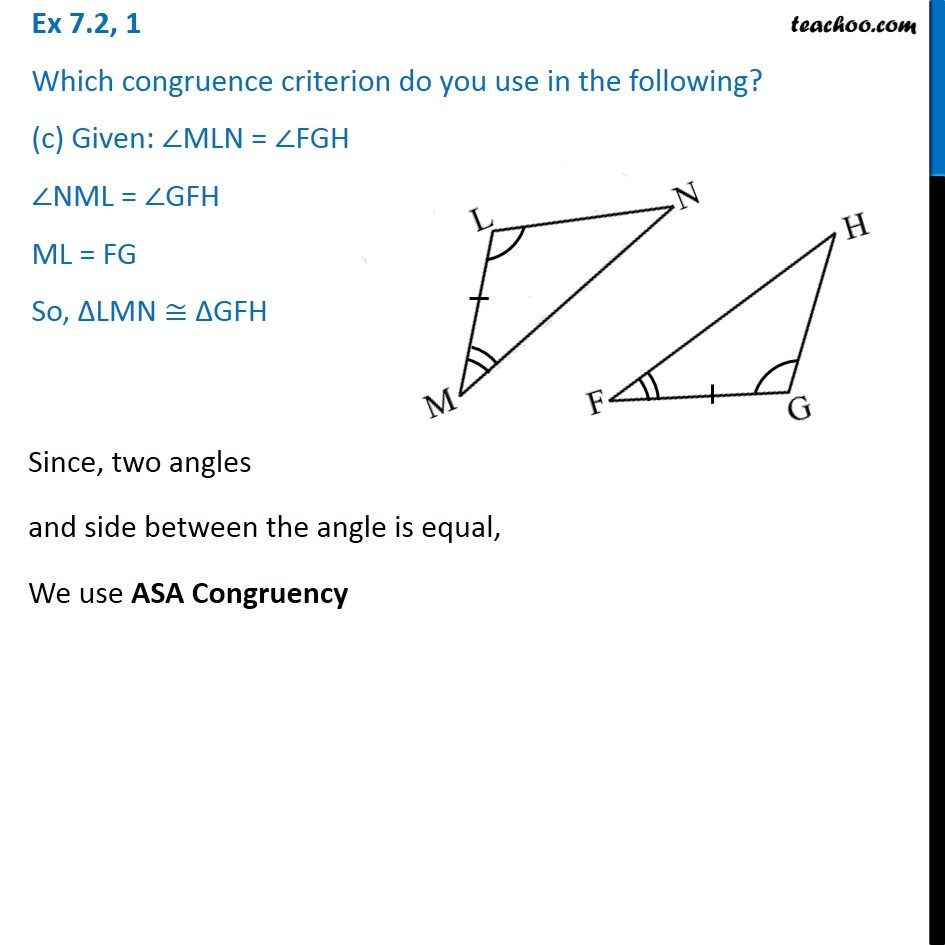Congruency - Different Criteria's

Congruence of Triangles
Serial order wiseLearn in your speed, with individual attention - Teachoo Maths 1-on-1 Class

### Transcript

Question 1 Which congruence criterion do you use in the following? (c) Given: ∠MLN = ∠FGH ∠NML = ∠GFH ML = FG So, ∆LMN ≅ ∆GFH Since, two angles and side between the angle is equal, We use ASA Congruency